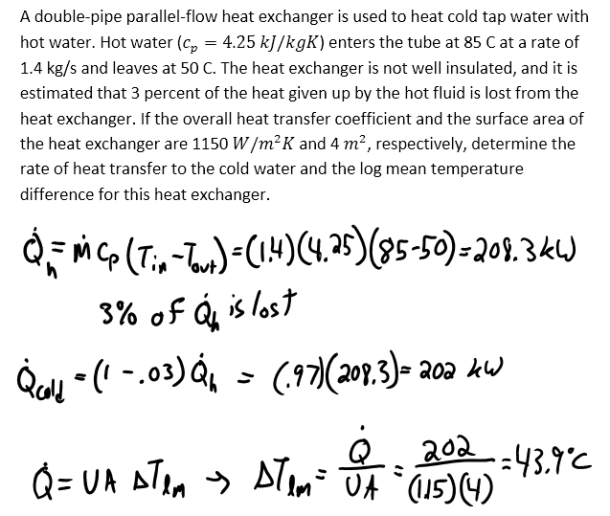heat and mass transfer problems engineering equations heat and mass transfer school homework engineering heat and mass transfer formulas heat and mass transfer solutions to heat and mass transfer problems full solution engineering problem solution heat and mass transfer math problems engineering equations heat and mass transfer school homework engineering solutions to heat and mass transfer formulas heat problems mass problem solutions to transfer problems full solution heat and mass transfer
heat and mass transfer problems engineering equations heat and mass transfer school homework engineering heat and mass transfer formulas heat and mass transfer solutions to heat and mass transfer problems full solution engineering problem solution heat and mass transfer math problems engineering equations heat and mass transfer school homework engineering solutions to heat and mass transfer formulas heat problems mass problem solutions to transfer problems full solution heat and mass transfer
Highalphabet Home Page heat and mass transfer problem solutions Heat and Mass Transfer Page
A double-pipe parallel-flow heat exchanger is used to heat cold tap water with hot water. Hot water (c_p=4.25 kJ/kgK) enters the tube at 85 C at a rate of 1.4 kg/s and leaves at 50 C. The heat exchanger is not well insulated, and it is estimated that 3 percent of the heat given up by the hot fluid is lost from the heat exchanger. If the overall heat transfer coefficient and the surface area of the heat exchanger are 1150 W/m^2 K and 4 m^2, respectively, determine the rate of heat transfer to the cold water and the log mean temperature difference for this heat exchanger.A double-pipe parallel-flow heat exchanger is used to heat cold tap water with hot water. Hot water (c_p=4.25 kJ/kgK) enters the tube at 85 C at a rate of 1.4 kg/s and leaves at 50 C. The heat exchanger is not well insulated, and it is estimated that 3 percent of the heat given up by the hot fluid is lost from the heat exchanger. If the overall heat transfer coefficient and the surface area of the heat exchanger are 1150 W/m^2 K and 4 m^2, respectively, determine the rate of heat transfer to the cold water and the log mean temperature difference for this heat exchanger.